Courses

NEET Previous Year Questions (2014-20): Classification of Elements & Periodicity in Properties Notes | Study Chemistry Class 11 - Class 11

Class 11: NEET Previous Year Questions (2014-20): Classification of Elements & Periodicity in Properties Notes | Study Chemistry Class 11 - Class 11

The document NEET Previous Year Questions (2014-20): Classification of Elements & Periodicity in Properties Notes | Study Chemistry Class 11 - Class 11 is a part of the Class 11 Course Chemistry Class 11.
All you need of Class 11 at this link: Class 11

Q.1. Identify the incorrect match.     (2020)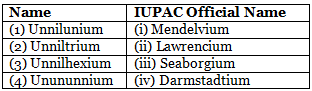(a) (3), (iii)
(b) (4), (iv)
(c) (1), (i)
(d) (2), (ii)

Ans: b
101 - Unnilunium - Mendelevium
103 - Unniltrium - Lawrencium
106 - Unnilhexium - Seaborgium
111 - Unununium - Roentgenium

Q.2. For the second period elements the correct increasing order of first ionisation enthalpy is:    (2019)
(a) Li < Be < B < C < N < O < F < Ne
(b) Li < B < Be < C < O < N < F < Ne
(c) Li < B < Be < C < N < O < F < Ne

(d) Li < Be < B < C < O < N < F < Ne
Ans:
b
‘Be’ and ‘N’ have comparatively more stable valence sub-shell than ‘B’ and ‘O’.
∴ Correct order of first ionisation enthalpy is:
Li < B < Be < C < O < N < F < Ne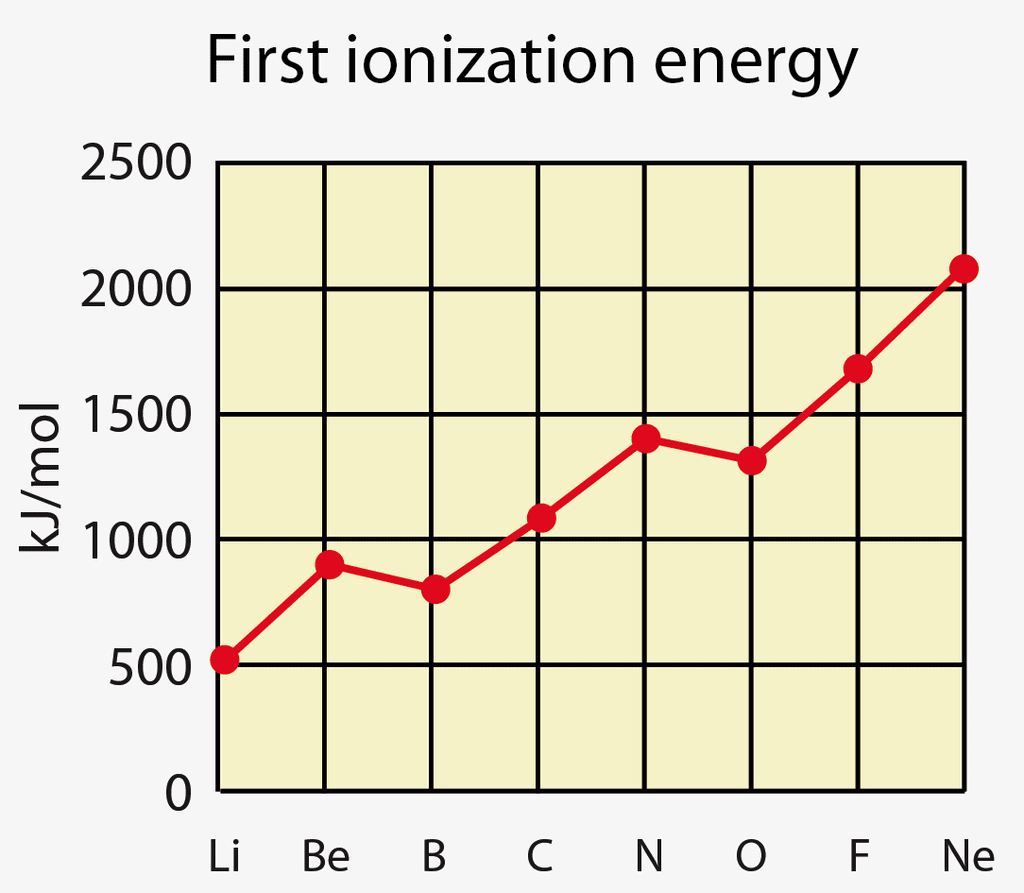Q.3. Magnesium reacts with an element (X) to form anionic compound. If the ground state electronic configuration of (X) is 1s2 2s2 2p3, the simplest formula for this compound is    (2018)
(a) Mg2X3
(b) MgX2
(c) Mg2X
(d) Mg3X2
Ans:
d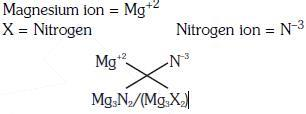Q.4. The element Z = 114 has been discovered recently. It will belong to which of the following family/groupand electronic configuration ?    (2017)
(a)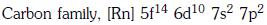(b)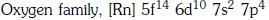(c)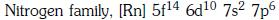(d)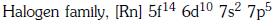Ans:
a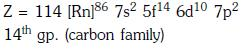Q.5. In which of the following options the order of arrangement does not agree with the variation of property indicated against it ?    (2016)
(a) Li < Na < K < Rb (increasing metallic radius)
(b) Al3+ < Mg2+ < Na+ < F- (increasing ionic size)
(c) B < C < N < O (increasing first ionization enthalpy)
(d) I < Br < Cl < F (increasing electron gain enthalpy)
Ans:
c and d
The correct order of increasing negative electron gain enthalpy is : I < Br < F < Cl and the correct order of increasing first ionisation enthalpy is B < C < O < N.

Q.6. The species Ar, K+ and Ca2+ contain the same number of electrons. In which order do their radii increase ?    (2015)
(a) K+< Ar < Ca2+
(b) Ar < K+< Ca2+
(c) Ca2+ < Ar < K+
(d) Ca2+ < K+ < Ar
Ans:
d
⇒ Ca2+ < K+ < Ar
Ar, K+ and Ca2+ are isoelectronic i.e with the same number of electrons, 18. For isoelectronic species, ionic radii decrease with increases in effective (relative) positive charge. Also Ar, K and Ca belong to the same period.

Q.7. Which of the following orders of ionic radii is correctly represented?    (2014)
(a) F- > O2- > Na+
(b) Al3+ > Mg2+ > N3-
(c) H- > H+ > H
(d) Na+ > F > O2-
(e) None

Ans:
e
Cations lose electrons and are smaller in size than the parent atom, whereas anions add electrons and are larger in size than the parent atom. Hence, the order is H- > H > H+.
For isoelectronic species, the ionic radii decreases with increase in atomic number i.e. nuclear charge. Hence, the correct orders are O2- > F- > Na+ and N3- > Mg2+ > Al3+.

There is no Question for NEET 2021

The document NEET Previous Year Questions (2014-20): Classification of Elements & Periodicity in Properties Notes | Study Chemistry Class 11 - Class 11 is a part of the Class 11 Course Chemistry Class 11.
All you need of Class 11 at this link: Class 11Use Code STAYHOME200 and get INR 200 additional OFF Use Coupon CodeChemistry Class 11

133 videos|238 docs|229 tests

Track your progress, build streaks, highlight & save important lessons and more!

,

,

,

,

,

,

,

,

,

,

,

,

,

,

,

,

,

,

,

,

,

;